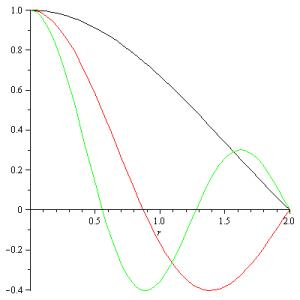Classroom Tips and Techniques: Eigenvalue Problems for ODEs - Part 2 - Maple Application Center
Application Center Applications Classroom Tips and Techniques: Eigenvalue Problems for ODEs - Part 2

## Classroom Tips and Techniques: Eigenvalue Problems for ODEs - Part 2This Application runs in Maple. Don't have Maple? No problem!
In this second of a three-part article, we use Maple to separate variables in a partial differential equation, then show how to obtain a solution of the resulting Sturm-Liouville eigenvalue problem. The PDE is Laplace's equation in a cylinder, and separation of variables leads to an eigenvalue problem involving Bessel's equation. We solve this singular Sturm-Liouville eigenvalue problem for the two cases of homogeneous Dirichlet and Neumann boundary conditions, and for the two cases of axial symmetry and asymmetry.

#### Application Details

Publish Date: June 25, 2007
Created In: Maple 10
Language: English

#### Share

This application is in the following Collection:

calculus

5